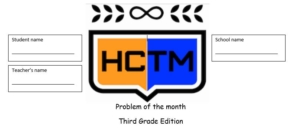Congratulations to the 3rd grade winner of the Problem of the Month competition for December! The winner is Nicholas from Ms. Steckman’s classroom at McKitrick Elementary.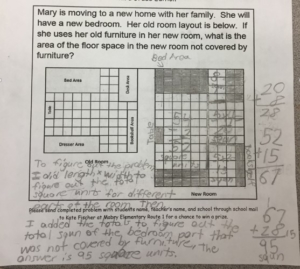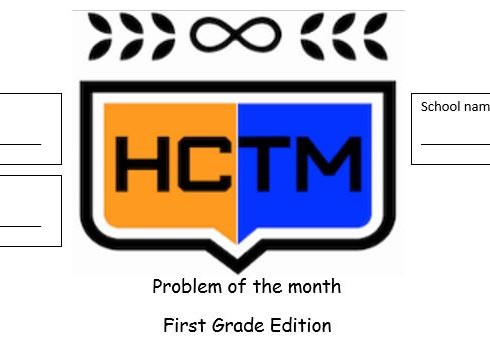Congratulations to the 1st grade winner of the Problem of the Month competition for December! The winner is Sagnik from Ms. Gordon’s classroom at Deer Park Elementary.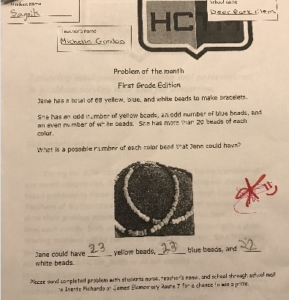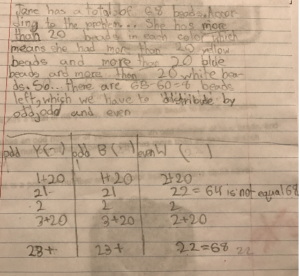Like most people, for my New Year resolution, I resolved to save more money!!! To accomplish this, I plan to save all my dimes in a Mason jar “bank.” My goal is to fill the mason jar completely!

Your task is help me determine how much money I will have saved if I fill the mason jar completely?

In the images below, you will see exactly one dollar in dimes in the jar.

According to the Standards for Mathematical Practices, students who, Make Sense of problems and persevere in solving them, “start by explaining to themselves the meaning of a problem and looking for entry points to its solution. They analyze givens, constraints, relationships, and goals. They make conjectures about the form and meaning of the solution and plan a solution pathway rather than simply jumping into a solution attempt.”

If I know that there is a dollar in the images above, what is the value of the dimes in the image below?

Questions to pose to students as they attempt to solve the problem:
What information do you need to solve this problem?
How are you going to use that information to help you solve?
How might knowing the first picture represents one dollar in dimes help you in solving the problem?
Is there more or less than 5 dollars in the jar right now? How do you know?

According to the SMP’s, “Mathematically proficient students check their answers to problems using a different method, and they continually ask themselves, ‘Does this make sense?'”

Now that you have some ideas about how to determine the value in the jar, I need to know, how much money will be in the jar if the jar is filled completely?

Happy Problem Solving!!!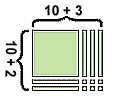MAFS.4.NBT.2.5 states that 4th graders should be able to:
Multiply a whole number of up to four digits by a one-digit whole number, and multiply two two-digit numbers, using strategies based on place value and the properties of operations Illustrate and explain the calculation by using equations, rectangular arrays, and/or area models.

When introducing multi-digit multiplication, the tendency is to dive right in to the standard algorithm. While the standard algorithm is an efficient strategy, it is very procedural and many kids copy performing the steps without understanding the process, which can result in misconceptions that are difficult to undo. A great approach for building conceptual understanding is to begin with base-ten models, and then connect those models to the partial products method and area model. This ensures that students have an understanding of all parts of the standard.

You can see from the following examples of 324 x 6 the similarities in the three methods. The area model is just a pictorial representation of the base-ten model, while partial products is a more abstract way of recording both strategies. All strategies are rooted in the student’s understanding of place value. The standard algorithm is actually a short-cut method of partial products, and fluency with the standard algorithm is a 5th grade standard. (https://www-k6.thinkcentral.com/content/hsp/math/hspmath/na/gr3-5/itools_intermediate_9780547274058_/basetenblocks.html virtual base-ten blocks were used to create the base-ten model.)Students see that to multiply 324 x 6, they actually multiply 6 times the ones, 6 times the tens, and 6 times the hundreds. These strategies allow students to break larger problems down so they can work with their basic facts and multiples of 10 and 100.

The area model is a great opportunity to make connections to arrays and students’ experience in 3rd grade with area.

All three strategies can also be applied when multiplying two 2-digit numbers, as shown in the representations of 12×13 shown below.
🙂If your students are struggling with using Think Addition to subtract, check out this video!Are your students still struggling with counting objects? Check out this quick video!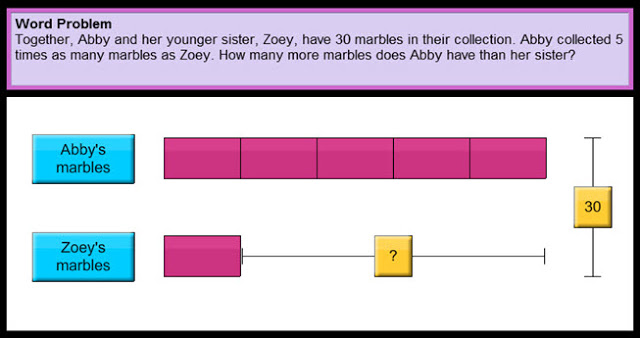Using simple bar models, often referred to as the “Singapore method”, to represent the mathematical relationships in comparison word problems helps students organize their thinking to more easily make sense of the problems and generate equations. Drawing bar models provides students with a strategy for visualizing abstract word problems in a more concrete way.

The videos below demonstrates how the bar model can be used to help make sense of multiplicative comparison problems. The tutorials use the “Thinking Blocks” virtual tool which can be found at http://www.mathplayground.com/thinkingblocks.html. This website has a variety of tutorials on modeling different types of comparison word problems, including those with two-steps. This tool not only has the benefits of supporting students with visualizing word problems, but it also provides them opportunities to manipulate models on the computer.

“>

“>Check out the video below for a quick and easy way to reinforce mathematics concepts on a number line.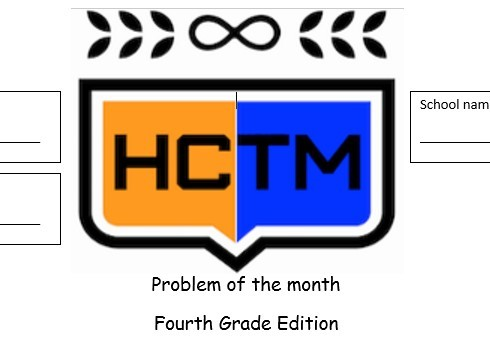What math do you see in this picture?According to the Standards for Mathematical Practices, “Mathematically proficient students make sense of quantities and their relationships in problem situations.”

How might students make sense of the quantities in this picture?
What math might they identify?

Happy Problem Solving!!!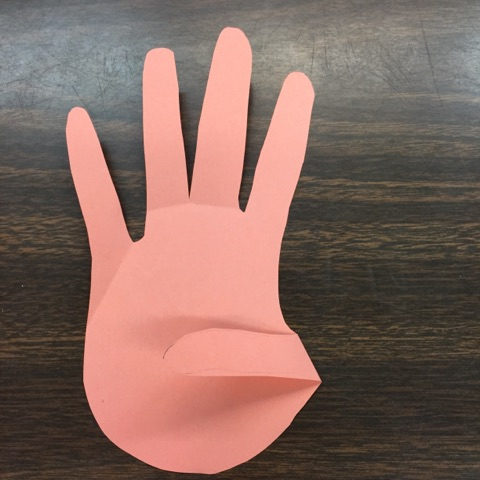Ahhh you had two weeks off for Winter Break and you are returning refreshed and ready to kick off the 2017 school year! Pretty soon 18 little minds will reenter your classroom! 17 days off of school means that it is an excellent time to reestablish classroom norms and expectations.

One way to review expectations while reinforcing number combinations is through the “Give me” strategy. Many teachers are familiar with the “Give me 5” strategy where students freeze and place their attention on the teacher or speaker.

A twist on this activity is for the teacher to say “Give me 5” but only hold up 3 fingers like in the image below.Students think about what other number combined with 3 makes 5. Since 2 is the addend that when combined with 3 makes a sum of 5, students should put 2 fingers in the air like in the image below.
***Note at this point in the year students have explored different combinations for making numbers, they have not formally been introduced to the idea that these number combinations are addends***To reinforce the number combinations ask students questions such as:
Why did you put up ___ fingers?
How did you know to put up ___ fingers?

This strategy could be extended to any number combination.
The teacher could say, “Give me 10” and hold up 1 finger in the air.Students would respond by holding up 9 fingers because the addends 1 and 9 make ten.
***Teacher Tip for managing materials: If you want students hands to be free of materials, select an addend that requires them to use both hands (any addend 6 or greater).Another twist on the strategy is for the teacher to hold up a sum and the students create the addends with their hands in the air. For example, a teacher might say, “What two numbers combined make 3 ?”If the sum is 3, students would hold up the addends 2 and 1Be creative!!! Think about different ways you can reinforce behavior and math at the same time!!!Are your students still struggling with One to one correspondence? Check out this quick Origo One video!

https://youtu.be/dvyLUpE6o7AFor information on using an area model to represent fractions, visit: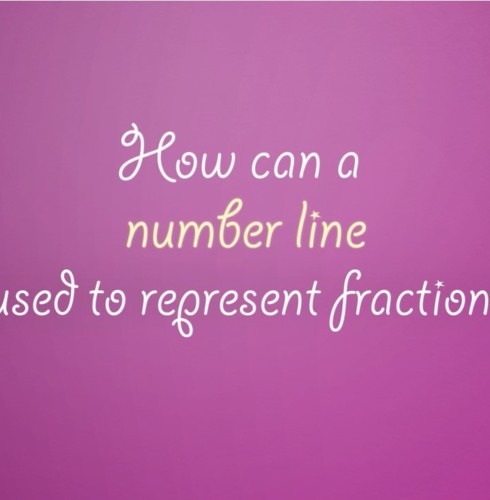For information on representing fractions on a number line visit:In 3rd grade students are expected to, “Understand a fraction as the quantity formed by 1 part when a whole is partitioned into equal parts.” The best way for students to develop this understanding is by putting fractions into real world context and create area models.

The Top 5 Things Students in 3rd Grade need to understand about modeling fractions with an area model are:

1. Students need to understand when it comes to modeling fractions with an area model, that the parts need to be equal or cover the same amount of space.

For example: A common misconception is shown below. Students need to understand that this is not demonstrating fourths because they are not equal parts.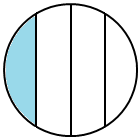2. Understand that the parts of the whole can be equal without having the same shapes within the whole. A great way to explore this is give students square post-it notes and have them find all the fourths they possibly can. From there – they can prove they are equal by cutting the parts up and comparing the size of the pieces.

The below shapes are common ways that students break squares into fourths because they are the same area and the same shape.We want students to understand that the below shape is also cut into fourths because they cover the same amount of space or are the same area.3. Name the equal parts found in the whole as halves, thirds, fourths, sixths and eighths. This occurred in 2nd grade using the language of fractions without using numbers to represent. You want to encourage students to identify shapes broken into each fractional amount.

The below shape is cut into sixths because there are six equal pieces that make up the whole.4. The numerator represents the number of pieces being discussed or focused on and the denominator represents the amount of total equal pieces in the whole. At this time students need to be able to represent it in fraction language and numerically as a fraction.

The picture below models 3/8 of the whole as shaded. The numerator 3 represents the number of equal pieces shaded. The denominator 8 represents the total number of equal pieces that make up the whole.5. Fractions can be found in more shapes than just squares or circles. Students need experiences with determining, creating and identifying fractions in various shapes to know that fractions can be found anywhere in the real world.

Triangle Example:Hexagon example: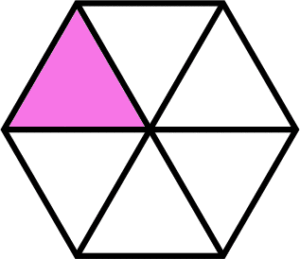Rectangle example with fraction tiles:We also want to eventually move students to drawing models of fractions. At this time, you want to encourage discussions about which wholes encourage more precise drawings for modeling specific denominators.

For example: Students are not as precise at drawing thirds in a circle as they are in a square.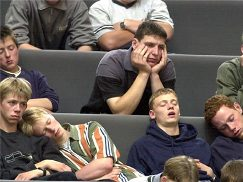Do your students look like they are filming a “mannequin challenge” when it comes to math interaction and discourse? Research has shown that children engaged and interacting with deep, purposeful tasks will have greater learning gains. So how can we get them interested?

Step one, “Burn your podium.” Check out this Edutopia article including tips on how to turn the focus and conversation over to the students.As I was driving home the other night I noticed this beautiful display of lights and it got me thinking about…Math!

Math is all around us….especially this time of year, so why not have a little fun with math in these winter months!

As you drive through town, I’m sure you may have noticed all the amazing winter displays. Have you ever wondered how many lights it really takes to create these dazzling decorations?

How might you determine the amount of lights you need?
What information would you need to know to solve this problem?

SMP 4, Modeling with Mathematics, requires students to apply the mathematics they know to solve problems arising in everyday life, society, and the workplace. There are plenty of opportunities to have our students engage in SMP 4 on a regular basis. This can be achieved by helping our students recognize the math in every day life.

It can be as simple as snapping a photo of something you find in the yard, such as acorns, and having your students generate an estimate!

Or as simple as determining how many ingredients you would need to triple a recipe to cook a holiday meal.1. What’s included when teaching area in 3rd grade?
• Solving real-world problems involving area of polygons
• Solve Real World Problems – Determining when it is necessary to find the area or perimeter from a given story problem.
• Recognize that area is comprised of square units
• Cover a space to determine the area without overlapping or gaps
• Measure area by counting unit squares
• Relate area to multiplication and addition (The formula for area is not addressed until 4th grade but they can relate to arrays for multiplication).
• Find the area of rectilinear figures (shapes with multiple rectangles).

2. Strategies for determining area:
• Give students a problem scenario where they determine which shape covers the most space before telling them there are using square units to determine area. This will help them discover and understand what the concept of area is.

Example: Tony was given the job of sectioning off part of the P.E. court for an assembly. He needs to find the design that will fit the most children. Which rectangle covers more space?• Counting square units
• Use addition/multiplication to determine the area

3. Relate Area to the Real World – Put tasks in real world context and make it relevant. Students should learn how area relates to them in their daily life. This will make it more meaningful and accessible for all students.

Example perimeter problem in real-world context: “Ms. Jones needs to tile this section of the classroom with new square tiles that don’t overlap and have no gaps. How could we determine how many tiles Ms. Jones will need to purchase?”(Note – to make the task even more relevant actually have students explore a given section in the classroom and problem solve).

4. Hands-on Exploration – Use the concrete-representational-abstract format to help students determine and understand the concept of area.

How do you make it happen? Be careful not to rush students to abstract. You want them to have a solid understanding of what area is through hands-on experiences and exploration. Here is a flow that should be utilized:
• Concrete – use manipulatives such as square tile and pentominoes to explore counting units around the outside of a figure to determine the perimeter.
• Representational – use graph paper and pictures to explore area on paper.
• Abstract – determine area of a figure by adding or multiplying. Encourage students to revisit the attributes of different quadrilaterals to make this happen, specifically rectangles and squares. Example: If I know one row in my figure has 5 square tiles and all the rows have the same amount, I can add 5+5+5+5 or multiply 4×5 to find the area of 20 square units.

Fun task to implement – give the students a given are or range of possible areas to look for and have them go around the classroom measuring objects to determine the mystery object(s). Could have them post-it notes or “square units” to determine the area.

Tools to use:
• Square tiles – to represent figures and count to determine area• Pentominoes – to determine area of irregular figures• Geoboards – to create or determine number of square units or area of given polygons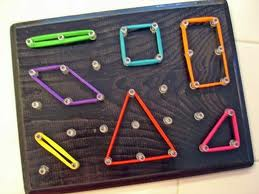• Graph paper – to represent figures and begin using more efficient strategies for determining the area• Rulers – to measure the length of figures to determine the area• Quick pictures – to draw the polygon and determine the area from a given problem

5. Precision with units – make sure students are being precise with their measurement units. Have them label the area in units each time they measure. Also have them compare units: “Would a figure with an area of 20 centimeters or 20 meters be larger?”

Consider using the following book title, “Bigger, Better, Best,” to explore the concept of area!